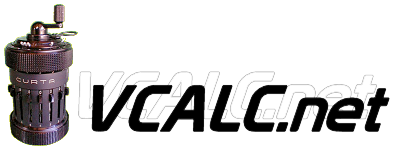# Early TI Calculators Accuracy

(since April 22, 1997)

Last Update: August 18, 2021 -- THE CURTA REFERENCE

Historical contribution from John H Meyers (jhmeyers@mum.edu)

For a couple of years, TI was the only calculator maker which used a wrong method for multiplication, causing the final digits of many products to be incorrect! For example, 1.9999999 * 4.9999999 happens to be exactly 9.99999930000001, so that any self-respecting "truncating" calculator giving 8 to 14 digits of result should have given the answer as 9.9999993; however, the TI Programmer (and *all* other TI models of that era having internal registers that kept nine significant digits and rounded the display to eight digits) would have displayed 9.9999987 or so! Other models which kept 11 internal digits (but still displayed only eight) also had this same fundamental bug, but the errors were usually concealed by the fact that the last three digits of the internal result, which were incorrect, remained unseen; you can nonetheless "see" the hidden incorrect digits by subtracting out the correct leading portion of the result (e.g. subtract 9.99999 in the above example), which will then disclose all the following digits (a final multiplication by, say 100000, may be necessary also, to shift all displayable digits into view).

All scientific models which used algorithms to derive other functions (log, trig, etc.) were likewise affected by the fact that every time they did a multiplcation as part of that algorithm, the answer came out a bit too small, producing a distinct tendency for "chained" results (e.g. 30 SIN INV SIN SIN INV SIN ...) to keep dropping in value.

My analysis of how TI did this feat is that instead of correctly starting with the least-significant multiplier digit, and then shifting rightward the fully-developed rightmost digits of what otherwise would have been a fully correct double-precision result as each successive more-significant multiplier digit was used, some hacker at TI decided to start with the most-significant multiplier digit, and then shift rightward the other factor, dropping off its least significant digit even before the second most-significant multiplier digit was used, etc.

Naturally, you can see immediately that the product of the least significant digits of each factor will never be included in this scheme; in fact many other of the "digit cross-products" are neglected as well. If you look at the numerical example I gave above, even the very least significant "9*9" product is essential for generating a "carry" which propagates all the way leftward into the eighth most-significant digit position of the correct answer, so obviously the result of the "hack" method is already clearly going to be incorrect. If you take into account the internal register widths actually used and the sum of all the "lost" digit cross-products, you successfully predict the exact wrong results which those TI calcs delivered for several years!

If you have any old LED models (starting from about when the very crummy old 5x8 standard keyboards were introduced), or even the SR-50 or some-era early LCD models (same keyboard!), try the above example to confirm this!

Rick Furr (rfurr@vcalc.net)
Back to The TI Calculator Page
Back to The Calculator Reference